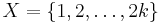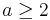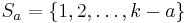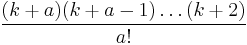# Solution Feb 12, 2012

Problem

Suppose X is a finite set such that | X | = 2k for some positive integer k. Suppose there's a family F of subsets of X, where each element of F has cardinality k, and such that every subset of X having cardinality k − 1 is uniquely contained in some element of F. Prove that k + 1 is prime.

Solution

Without loss of generality let$X = \{1,2,\ldots, 2k \}$. For$a \geq 2$, I claim that the number of sets in F containing$S_a = \{1,2,\ldots, k-a\}$ is precisely$\frac{(k+a)(k+a-1)\ldots(k+2)}{a!}$.

There are 2k − (ka) = k + a numbers in XSa. Choosing any a − 1 numbers in XSa and including them in Sa will provide us with a subset of cardinality k − 1, which, by the condition on F, is uniquely contained in some set in F. There are clearly a! repetitions in creating a set in this manner, so the claim is proved.

Since$\frac{(k+a)(k+a-1)\ldots(k+2)}{a!}$ is integer for all$a \geq 2$, it's now easy to see that k + 1 is prime.